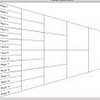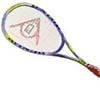#### You may also like### Rain or Shine

Predict future weather using the probability that tomorrow is wet given today is wet and the probability that tomorrow is wet given that today is dry.### Knock-out

Before a knockout tournament with 2^n players I pick two players. What is the probability that they have to play against each other at some point in the tournament?### Squash

If the score is 8-8 do I have more chance of winning if the winner is the first to reach 9 points or the first to reach 10 points?

# Magic Bag

##### Age 16 to 18Challenge Level

Although this problem might sound insoluble with the information given, the magic bag assures us that there is a nice answer, as does Mr Cox from North Berwick High School who solved this problem very nicely (see his solution here) so let us proceed!

Suppose that there are $b$ black balls and $w$ white balls in the bag, and that there are at least two of each (we can think about the other cases at the end).

Then
$$\begin{eqnarray} P(bb) &=& \frac{b}{b+w}\cdot\frac{b-1}{b+w-1}\cr P(wb) &=& \frac{b}{b+w}\cdot\frac{w}{b+w-1}\cr P(bw) &=& \frac{w}{b+w}\cdot\frac{b}{b+w-1}\cr P(ww) &=& \frac{w}{b+w}\cdot\frac{w-1}{b+w-1} \end{eqnarray}$$
Although these look complicated, the denominators in each case are the same.

Since the probabilities are mutually exclusive we know that
$$P(bb)+P(ww) = 0.5$$
Thus,
$$2b(b-1)+2w(w-1) = (b+w)(b+w-1)$$
Expanding and rearranging gives us
$$b^2-b+w^2-w-2wb=0$$
So, where are we now? We've applied the probability and are left with an expression involving $w$ and $b$. This can be factorised as
$$(b-w)^2=b+w$$
Some thought should convince you that this expression is satisfied if $b$ and $w$ are consecutive triangle numbers. But we need to ask: are there any other solutions? We need to ask this because for any choice of $w$ there will be up to $2$ solutions for $b$ upon solving the equation. The quadratic formula tells us that for any given $w$
$$b=\frac{1+2w\pm \sqrt{1+8w}}{2}$$
Now, we need to impose the condition that $b$ and $w$ are natural numbers. This would require that $1+8w$ is a square number, say $N^2$. Then
$$w =\frac{N^2-1}{8}=\frac{(N+1)(N-1)}{8}$$
For $w$ to be a natural number, $N$ must be an odd number, which means that $(N+1)$ and $(N-1)$ are consecutive even numbers, which means that $\frac{N+1}{2}$ and $\frac{N-1}{2}$ are consecutive natural numbers. Thus, $w$ is half the product of two natural number which means that $w$, by definition must be triangular. Thus there is a solution in this case if and only if $w$ and $b$ are consecutive triangle numbers bigger than $1$.

Finally, we consider the special cases where there are less then two of each ball.

Clearly, the balls cannot all be the same colour, as then the chance of drawing two balls of the same colour would be $1$. Clearly, there cannot be exactly one ball of each colour, as then the chance of drawing two balls of the same colour would be $0$. If there were exactly one black ball and $w> 1$ white balls then the chance of drawing two balls of the same colour would be
$$\frac{w}{w+1}\cdot\frac{w-1}{w} = 0.5$$
This has exactly the two solutions $w=0, 3$. Since $3$ is also triangular, our rule still holds.

Putting this all together proves that magic bag can, and only can, contain consecutive triangle numbers of white and black balls.